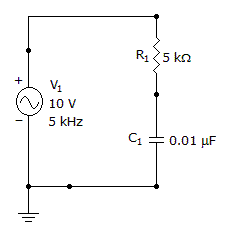# Electronics - Capacitors - Discussion

### Discussion :: Capacitors - General Questions (Q.No.53)

53.

What is the impedance of this circuit?[A]. 5928[B]. 2000[C]. 1902[D]. 1798Explanation:

Impedance Cannot be Directly Measured, We Need to find Xc first.

So,

Xc = 1/2πfC.

Xc = 1/( 2 x 3.14 x 5000 x 0.01 x 10-6 ).

Xc = 3184.7.

Z = Sqrt( Xc2 + R2 ).

Z = Sqrt( 3184.72 + 50002 ).

Z = 5928 Ω.

 Vignesh said: (Sep 8, 2013) Frequency = 1KHz in schematic diagram, but it has been substituted as 5KHz while solving. How?

 Ankur Tyagi said: (Nov 24, 2013) X= Sqrt(R^2+ Xc^2). It Must be more than 5 Kohm.